﻿ 基于T-S型模糊加权的多模软切换的风电机组变桨控制
«上一篇文章快速检索 高级检索

 智能系统学报2018, Vol. 13Issue (4): 625-632  DOI: 10.11992/tis.2017100090

### 引用本文HOU Tao, ZHANG Qiang. Multi-mode soft-switch variable-pitch control of wind turbines based on T-S fuzzy weighting[J]. CAAI Transactions on Intelligent Systems, 2018, 13(4), 625-632. DOI: 10.11992/tis.201710009.### 文章历史

Multi-mode soft-switch variable-pitch control of wind turbines based on T-S fuzzy weighting
HOU Tao, ZHANG QiangSchool of Automation and Electrical Engineering, Lanzhou Jiaotong University, Lanzhou 730070, China
Abstract: Variable-pitch control is an effective technique for ensuring the constant-power operation of wind turbines over the range of rated wind speeds. The frequent and strong action of a pitch actuator increases the mechanical fatigue load of wind turbines, affects the output quality of the generator, and reduces the service life of wind turbines. Most current switching methods switch only at a certain threshold, which causes switch oscillation. To address these issues, we propose a multi-mode soft switching variable-pitch control strategy based on Takagi-Sugeno (T-S) fuzzy weighting. In this method, intelligent and traditional controls are combined, based on the deviation of the real-time rotation speed of the generator from its rated rotation speed and the change rate of the real-time rotation speed. T-S fuzzy inference is also utilized to achieve a smooth transition in the output of the multi-mode controller by the use of fuzzy control, fuzzy adaptive proportional-integral-derivative control, and proportional-integral control to realize a soft switch. This method simultaneously employs the advantages of three kinds of control methods and solves the switch oscillation problem. In this study, we built a multi-mode soft-switch control model to address the variable pitch of permanent-magnet direct-drive wind turbines. The simulation results show that this method exhibits the advantages of the three control methods, overcomes switch oscillation, slows frequent action of the actuator, smooths the adjustment of the pitch angle, improves the precision of the output power, and reduces fluctuation.
Key words: wind turbines    electric variable pitch    T-S fuzzy weighting    multi-mode switching control    soft switch    switch oscillation    multi-mode    pitch control

1 风电系统数学模型 1.1 风速模型

 ${v_{{w}}} = {v_{{b}}} + {v_{{g}}} + {v_{{r}}} + {v_{{n}}}$ (1)
 ${v_{{b}}} = l \cdot \varGamma (1 + 1/s)$ (2)
 ${v_{\rm{r}}} = \left\{ \begin{array}{l} {\rm{ }}0,\quad{\rm{ 0 < }}t{\rm{ < }}{t_{{\rm{1r}}}},{\rm{ }}t \geqslant {t_{{{2r}}}} + {t_{{r}}} \\ {v_{{\rm{rmax}}}}(1 -\displaystyle \frac{{t - {t_{{{2r}}}}}}{{{t_{{{1r}}}} - {t_{2r}}}}){\rm{ }},\quad{t_{{{1r}}}} \leqslant t < {t_{{{2r}}}} \\ {v_{{\rm{rmax}}}},\quad{\rm{ }}{t_{2{{r}}}} \leqslant t < {t_{{{2r}}}} + {t_{{r}}} \\ \end{array} \right.$ (3)
 ${v_{{g}}} = \left\{ \begin{array}{l} 0,\quad{\rm{ 0 < }}t{\rm{ < }}{t_{{{1g}}}}{\rm{, }}t \geqslant {t_{{{1g}}}} + {t_{{g}}} \\ \displaystyle\frac{{{v_{{\rm{gmax}}}}}}{2}[1 - {\rm{cos}}(2{\text{π}}\frac{{t - {t_{1g}}}}{{{t_{1g}} - {t_{{g}}}}})],\quad{\rm{ }}{t_{1g}} \leqslant t < {t_{1g}} + {t_{g}} \\ \end{array} \right.$ (4)
 ${v_{{n}}} = 2\sum\limits_{i = 1}^n {\sqrt {{S_{\rm{r}}}({\omega _{{i}}})\Delta {\omega _{\rm{0}}}} } \cos ({\omega _{{i}}}t + {\phi _{{i}}})$ (5)

1.2 风力机数学模型

 $\left\{ \begin{array}{l} {P_{{m}}}{\rm{ = }} \displaystyle\frac{1}{2}{\text{π}} \rho {R^2}{C_{\rm{P}}}(\beta ,\lambda ){v^3} \\ {T_{{m}}} = {P_{{m}}}/{\omega _{{f}}} = \displaystyle\frac{1}{2}{\text{π}}{C_{\rm{P}}}(\beta ,\lambda ){v^2}/\lambda \\ \lambda = R{\omega _{{f}}}/v \\ {C_{{P}}} = 0.517\;6(116{\lambda _1} - 0.4\beta - 5){{\rm{e}}^{ - 21/{\lambda _1}}} + 0.006\;8{\lambda _1} \\ \end{array} \right.$ (6)

1.3 变桨执行机构数学模型

 ${G_{{\rm{pitch}}}}(s) = \frac{{\beta (s)}}{{{\beta _{{\rm{ref}}}}(s)}} = \frac{1}{{{\tau _{{b}}}s + 1}}$ (7)

2 基于T-S型模糊加权的多模变桨控制策略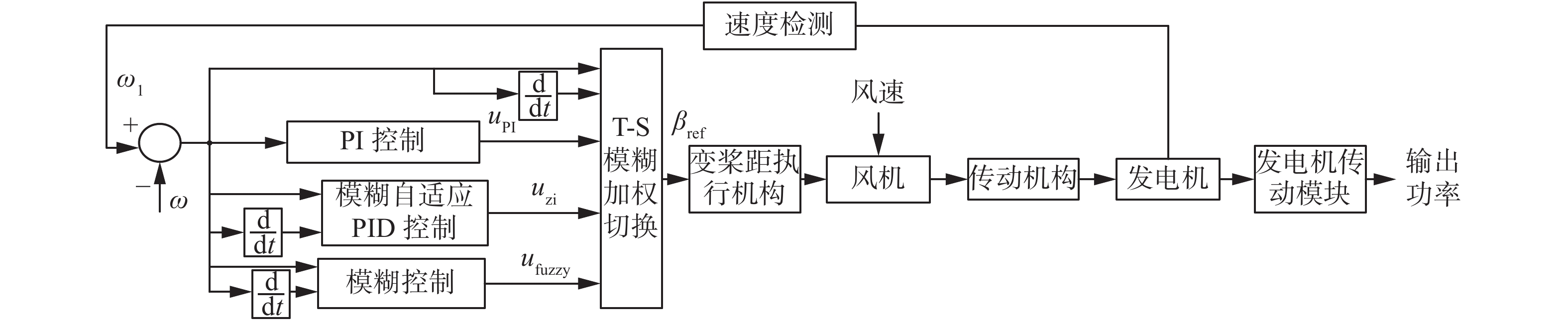Download: 图 1 T-S模糊加权变桨距控制框图 Fig. 1 Block diagram of T-S fuzzy weighted variable pitch control
2.1 模糊控制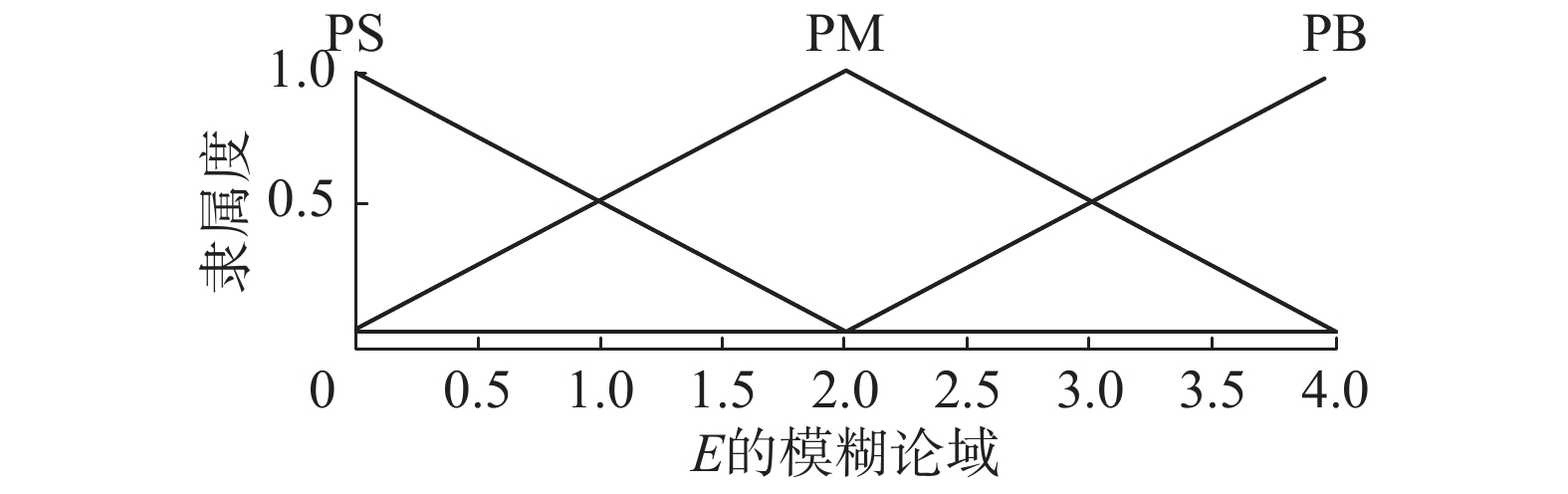Download: 图 2 E的隶属函数 Fig. 2 The membership of E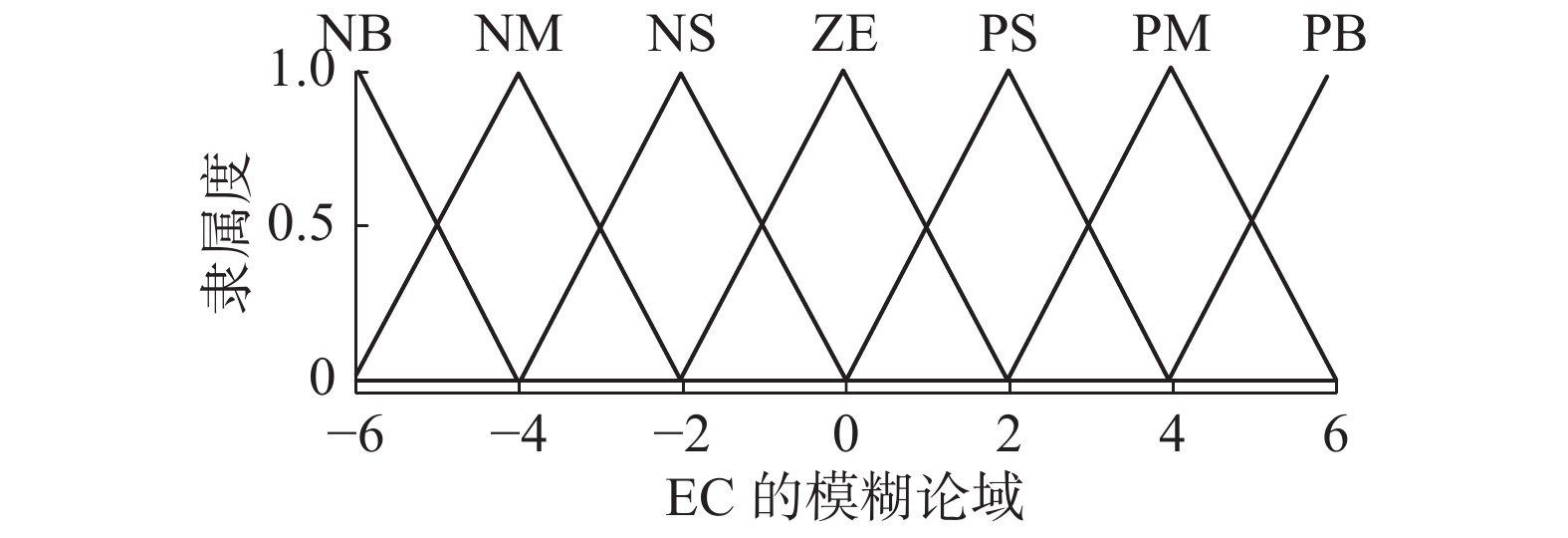Download: 图 3 EC的隶属函数 Fig. 3 The membership of EC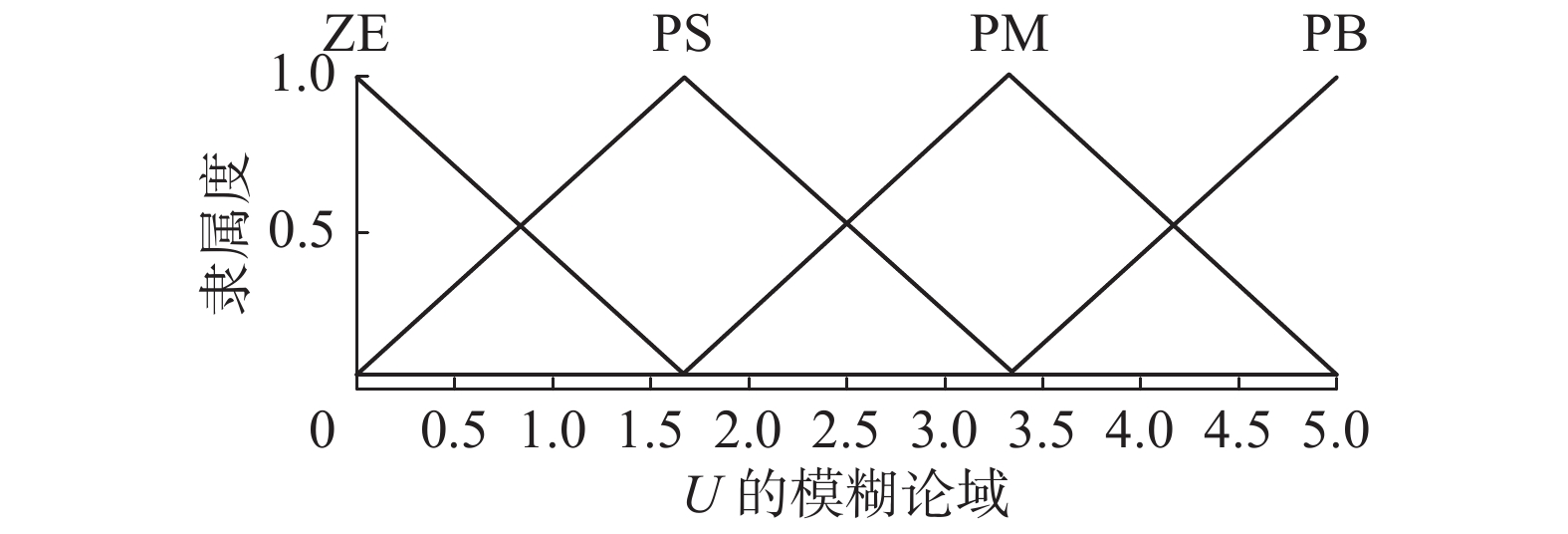Download: 图 4 U的隶属函数 Fig. 4 The membership of U表 1 ${U_{{\rm{fuzzy}}}}$ 的模糊控制规则 Tab.1 The fuzzy control rule of ${U_{{\rm{fuzzy}}}}$
2.2 模糊自适应PID控制表 2 $\Delta {k_{\rm{P}}}$ 的模糊控制规则 Tab.2 Fuzzy control rule of $\Delta {k_{\rm{P}}}$表 3 $\Delta {k_{\rm{I}}}$ 的模糊控制规则 Tab.3 Fuzzy control rule of $\Delta {k_{\rm{I}}}$表 4 $\Delta {k_{\rm{D}}}$ 的模糊控制规则 Tab.4 Fuzzy control rule of $\Delta {k_{\rm{D}}}$
2.3 PI控制

PI控制是PID控制的一种特例，当系统的偏差较小时，采用PI控制可以使控制系统具有较好的稳态性能。

3 基于T-S型模糊加权的多模软切换设计 3.1 设计原理

T-S型是一种适于表达复杂系统的动态特性，其输出的隶属函数可以是线性的或者是单点常数。本文主要利用T-S型模糊推理的隶属函数为单点常数使得其输出为单点常数这一特点来实现软过渡。区别于传统的切换方法只在某一阈值进行切换，本文引入了偏差及其导数，在到达阈值的前几个时刻，此时偏差小于设定的阈值，若偏差的变化率较大，本文使用的方法就进行了模态切换；同样，在到达偏差的后几个时刻，此时偏差大于设定的阈值，若偏差的变化率较小，本文使用的方法就不进行模态切换；具体的切换时刻由T-S型模糊推理实现，这也充分利用了模糊控制智能化的优势。T-S型模糊加权多模切换设计的目的是利用T-S型模糊推理平滑多种控制模态的输出，T-S型模糊加权切换的输出为模态的权值。其基本思想是根据系统的转速误差信息，需要在3个模态之间进行切换时，引入转速偏差和其导数进行模态切换判断，应用T-S型模糊推理方法，清晰化采用加权平均法，得到切换过程中3个模态的权值，使用加权求和法将3个模态的权值加权到3个模态的输出，从而平滑控制器的输出，实现T-S型模糊加权多模软切换。

${{\rm{MD}}_1}$ ${{\rm{MD}}_2}$ ${{\rm{MD}}_3}$ 分别为模糊控制模态、模糊自适应PID控制模态和PI控制模态； ${w_{{a}}}$ ${w_{{b}}}$ ${w_{{c}}}$ 分别为模糊控制模态的权值、模糊自适应PID控制模态的权值和PI控制模态的权值； ${U_{{\rm{fuzzy}}}}$ ${U_{{\rm{zi}}}}$ ${U_{{\rm{PI}}}}$ 分别为模糊控制、模糊自适应PID控制和PI控制的输出控制量， ${U_{{\rm{sum}}}}$ 为T-S型模糊加权多模切换的合成输出值。在大偏差时切换到模糊控制起主要控制作用，模糊自适应PID控制和PI控制起微小的控制作用，此时 ${w_{{a}}}$ 为主导权值；在小偏差时系统将很快趋于稳态，切换到PI控制起主要控制作用，模糊控制和模糊自适应PID控制起微小的控制作用，此时 ${w_{{c}}}$ 为主导权值；中等偏差时切换到模糊自适应PID控制，模糊控制和PI控制起微小的控制作用，此时 ${w_{{b}}}$ 为主导权值。把 ${w_{{a}}}$ ${w_{{b}}}$ ${w_{{c}}}$ 分别加权到 ${U_{{\rm{fuzzy}}}}$ ${U_{{\rm{zi}}}}$ ${U_{{\rm{PI}}}}$ ,即可实现多模加权软切换控制。T-S型模糊加权多模软切换设计包括4个步骤，分别为模糊化、模糊推理、去模糊化和合成输出量。

3.2 模糊化

T-S型模糊加权算法的模态划分取决于发电机的转速与其额定转速的偏差E及其变化率EC，利用这两个量来确定T-S型模糊推理各模态的权值。由于风速处于额定风速以上，此时转速高于额定转速，偏差为正，故采用单边模糊控制。选取发电机的转速与其额定转速的偏差E和偏差变化率EC的论域和模糊论域同前文模糊控制一样，E的模糊子集为 $\left\{ {{\rm{PS,PW,PM,PB}}} \right\}$ ，EC的模糊子集为 $\left\{ \rm{NB,NS,ZE,PS,PB} \right\}$

3.3 模糊推理表 5 模态模糊规则描述 Tab.5 The description of modal fuzzy rule
3.4 去模糊化

T-S型模糊控制的去模糊化采用加权平均法，设第 $i$ 条规则输出的结果为 ${u_{{i}}}$ ，它的权重为 ${w_{{i}}}$ $m$ 为规则数，则总输出为

 $U = \frac{{\displaystyle\sum\limits_{i = 1}^m {{w_{i}}{u_i}} }}{{\displaystyle\sum\limits_{i = 1}^m {{w_{{i}}}} }}{\rm{ = }}\frac{{{w_1}{u_1} + {w_2}{u_2} + \cdots {w_{{m}}}{u_{{m}}}}}{{{w_1} + {w_2} + \cdots {w_{{m}}}}}$ (8)

3种模态的权值 ${w_{\rm{a}}}$ ${w_{\rm{b}}}$ ${w_{\rm{c}}}$ 由式(8)计算得出。设定T-S型模糊模型的3个模态输出隶属函数为单点值，其值均为0和1，合理的设置模糊规则，使 ${w_{{a}}}$ ${w_{{b}}}$ ${w_{{c}}}$ 根据偏差及其变化率经过模糊推理和清晰化后其输出都为 $[0,1]$ 的精确值，在任意时刻， ${w_{{a}}}$ ${w_{{b}}}$ ${w_{{c}}}$ 的其中一个数值较大，另外两个数值较小，且满足：

 ${w_a} + {w_b} + {w_c} = 1$ (9)
3.5 T-S型模糊切换合成输出

 ${U_{{\rm{sum}}}} = {w_{{a}}} \times {U_{{\rm{fuzzy}}}} + {w_{{b}}} \times {U_{{\rm{zi}}}} + {w_{{c}}} \times {U_{{\rm{PI}}}}$ (10)
4 仿真分析与验证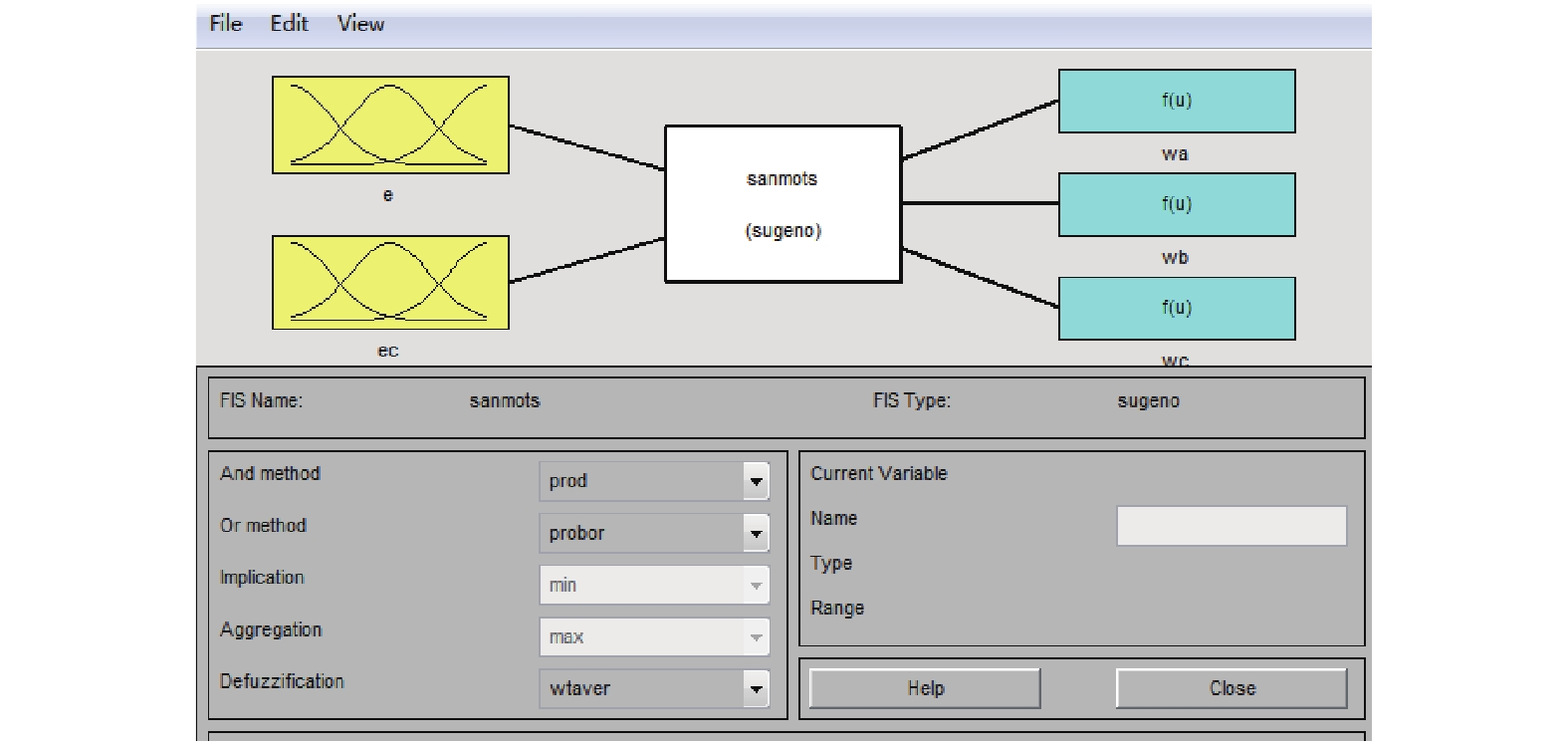Download: 图 5 T-S型模糊推理系统 Fig. 5 The T-S fuzzy inference system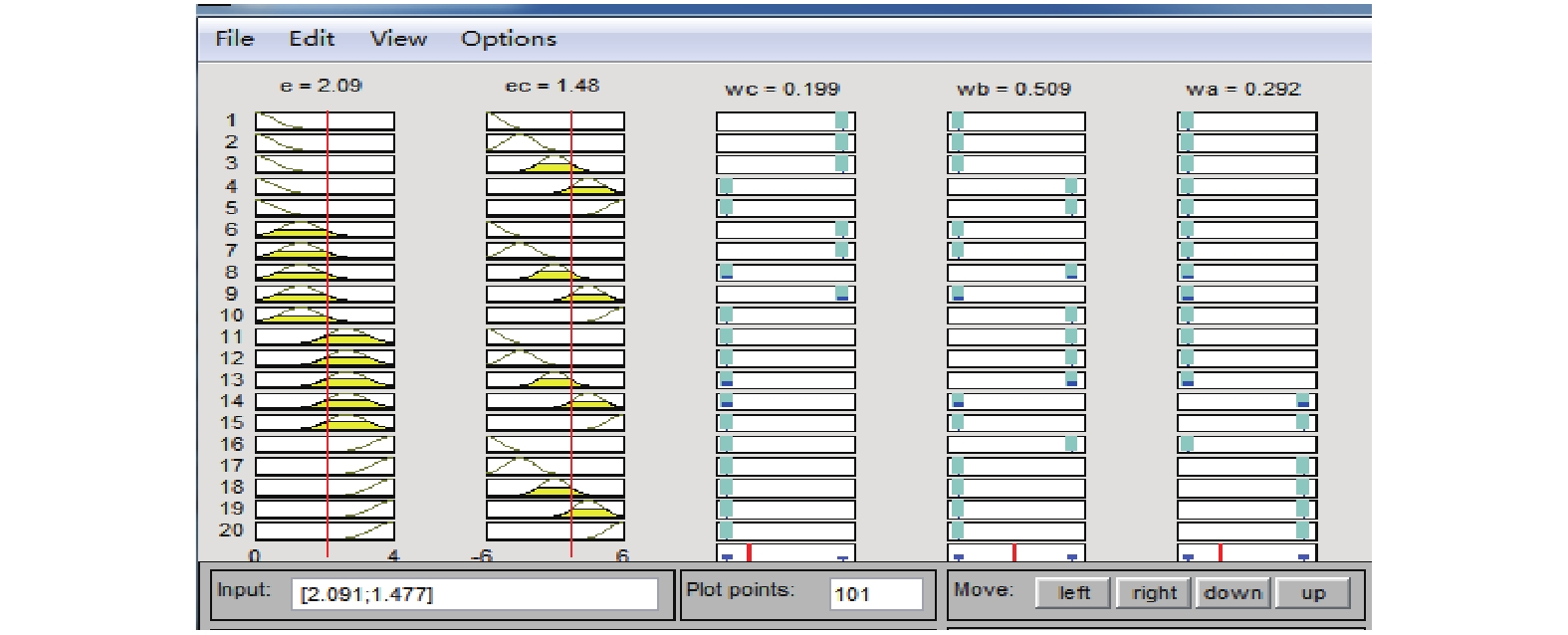Download: 图 6 ruler viewer中的权值输出 Fig. 6 The weight outputs in ruler viewer

1) 阶跃风速

0～5 s风速为 $13\,\, {\rm{m/s}}$ ，5 s时跃变为 ${\rm{18 \,\, m}}/{\rm{s}}$ 。风速如图7所示，4种控制方法下转速对比曲线如图8所示，桨距角对比曲线如图9所示，功率对比曲线如图10所示，T-S型模糊推理的权值输出如图11所示。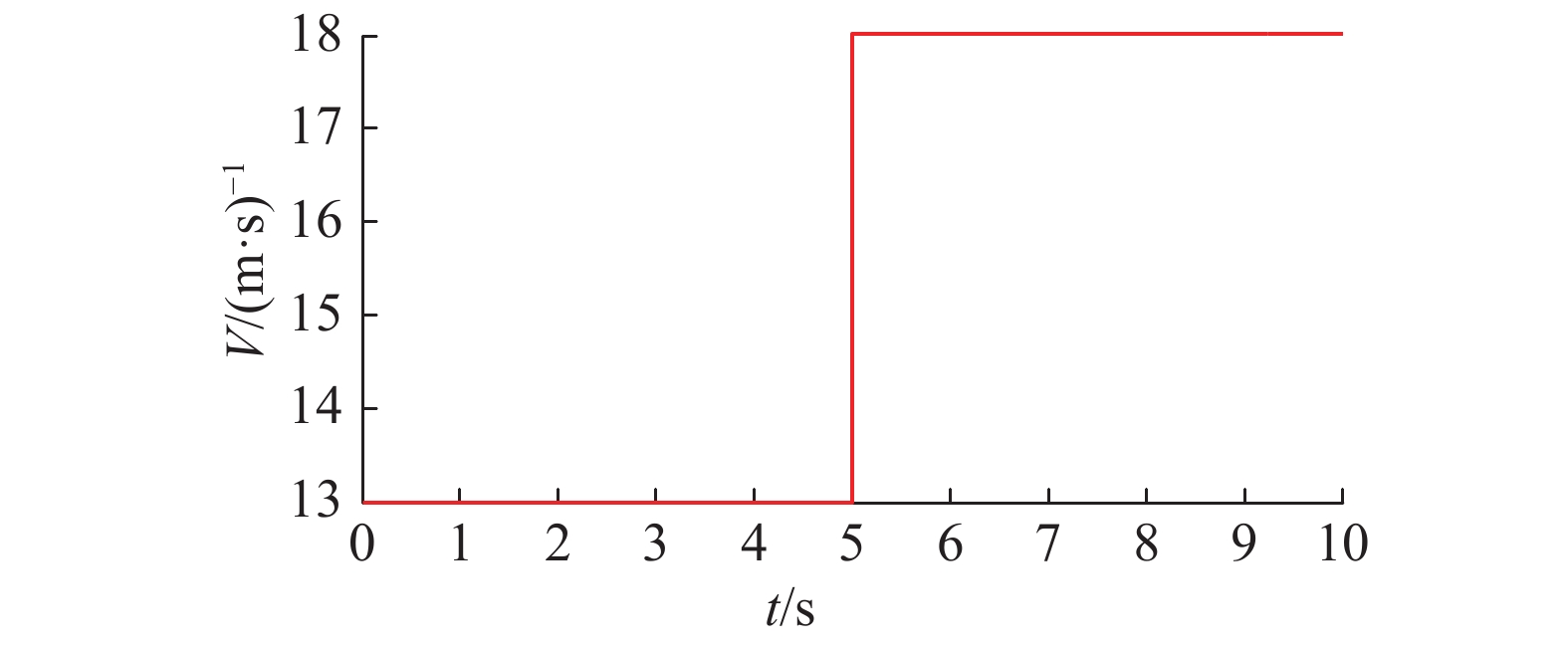Download: 图 7 风速 Fig. 7 The curve of wind speed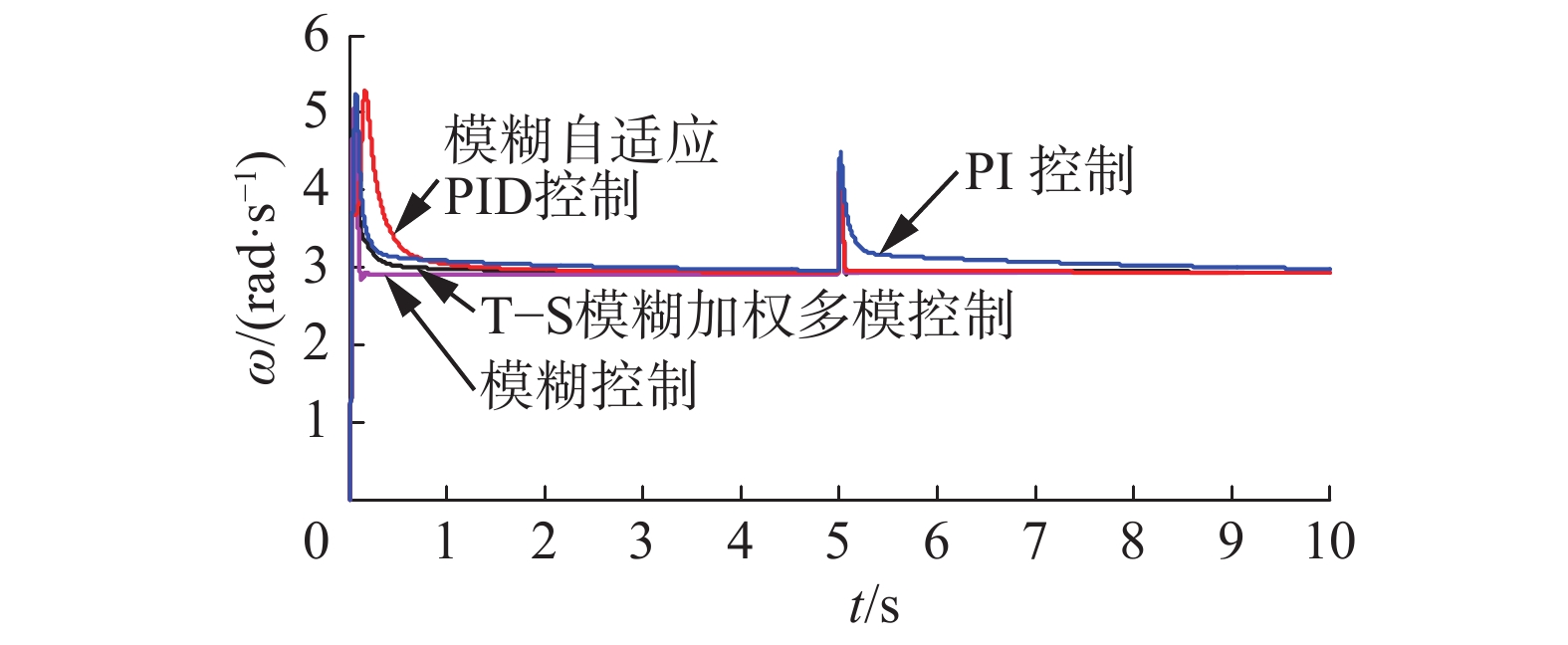Download: 图 8 转速对比 Fig. 8 The comparison of rotor speed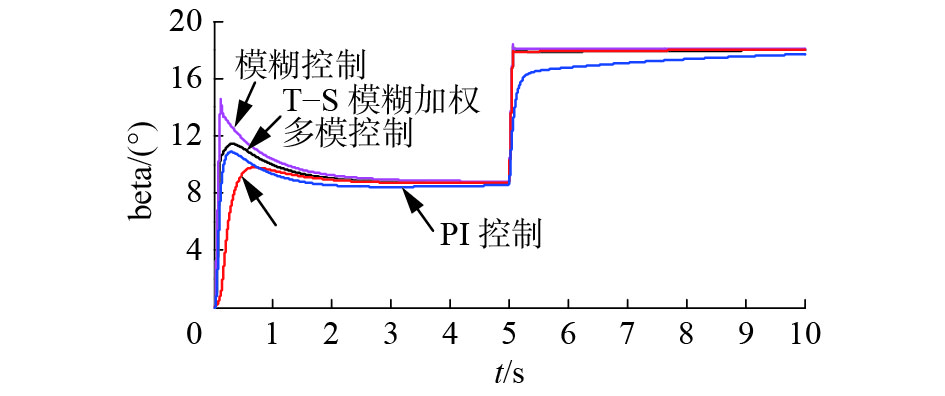Download: 图 9 桨距角对比图 Fig. 9 The comparison of pitch angle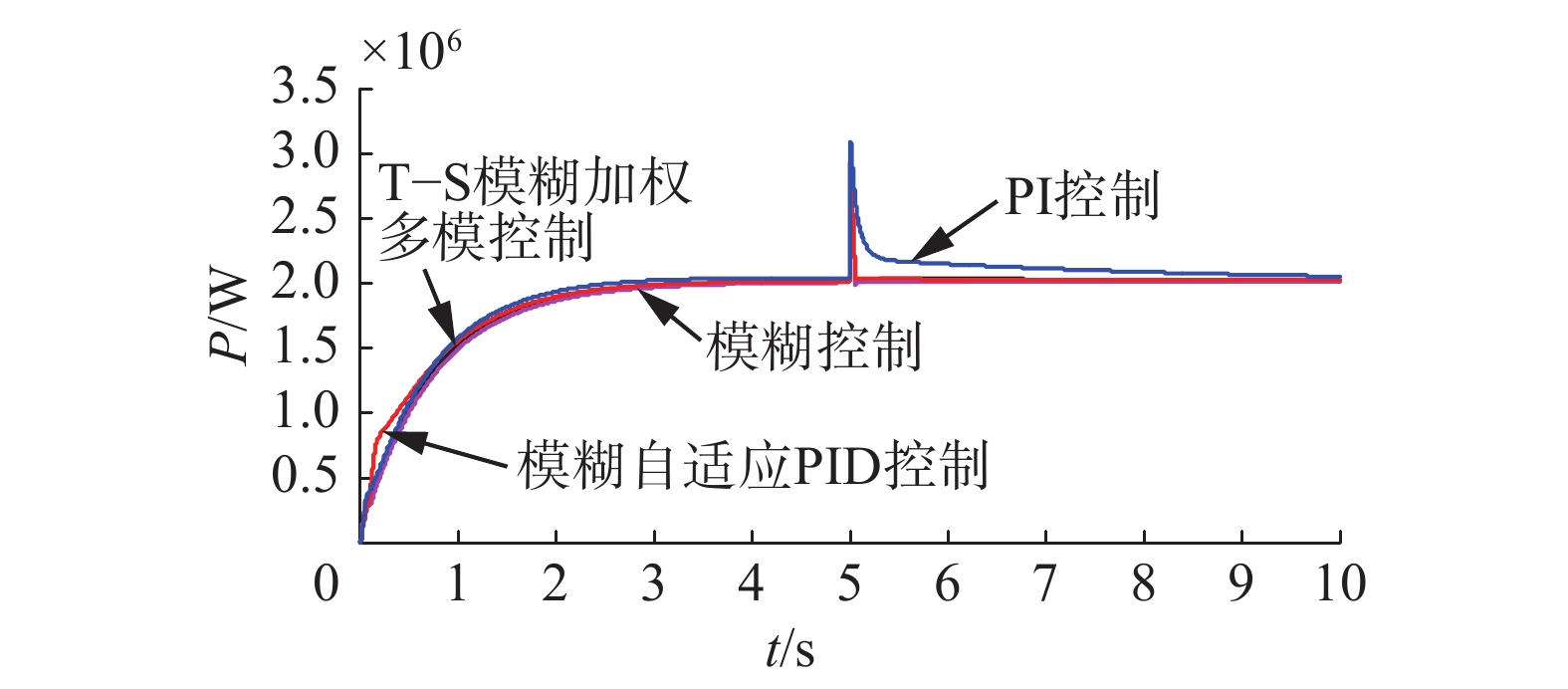Download: 图 10 输出功率对比 Fig. 10 The comparison of generator output power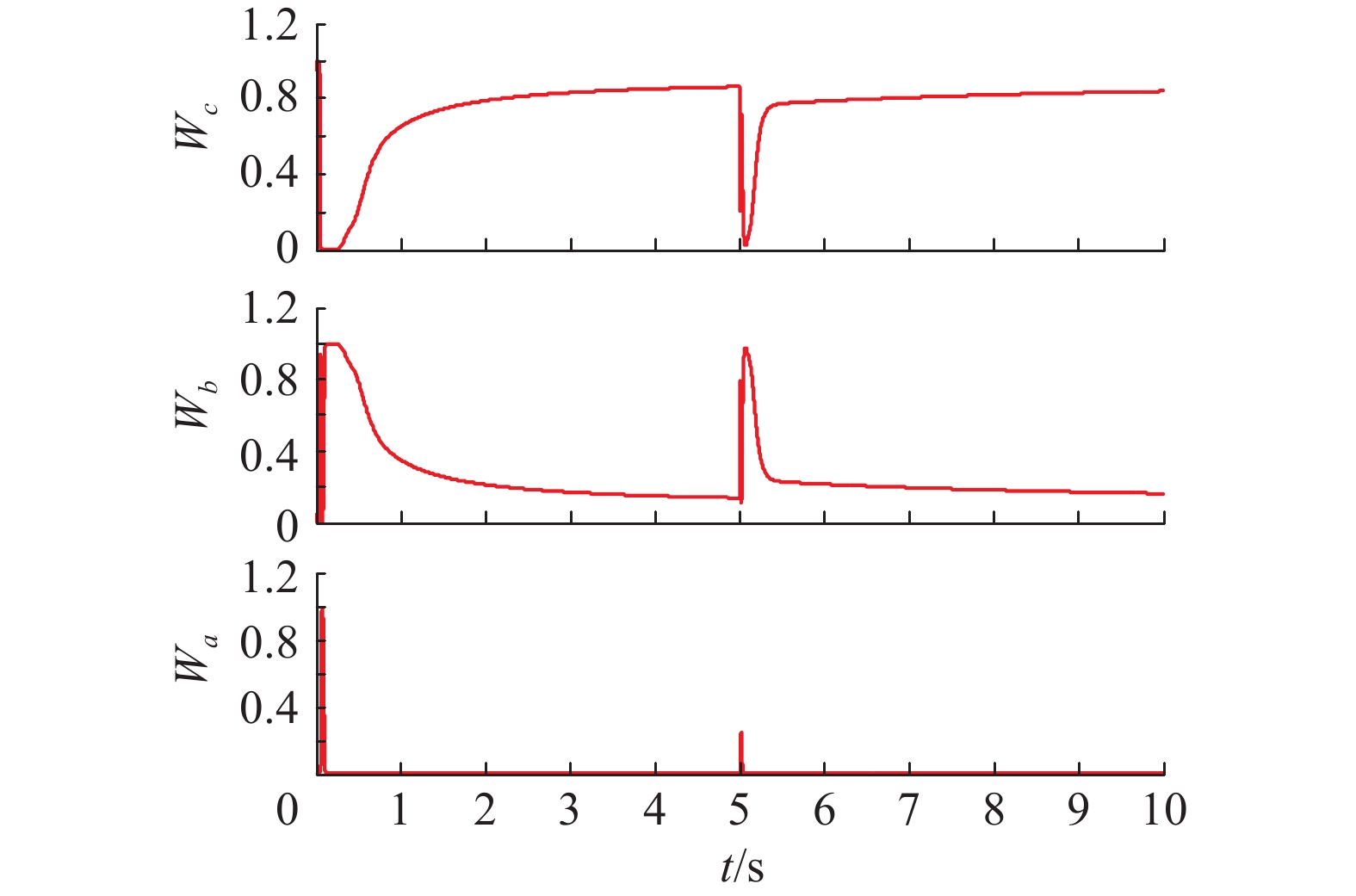Download: 图 11 T-S型模糊推理的权值输出 Fig. 11 The weight output of T-S fuzzy inference

2)组合风速

 ${v_{{n}}} = {v_{n{\rm{max}}}} \cdot {R_{{\rm{am}}}} \cdot \cos ({\omega _{{i}}} + {\phi _{{i}}})$ (11)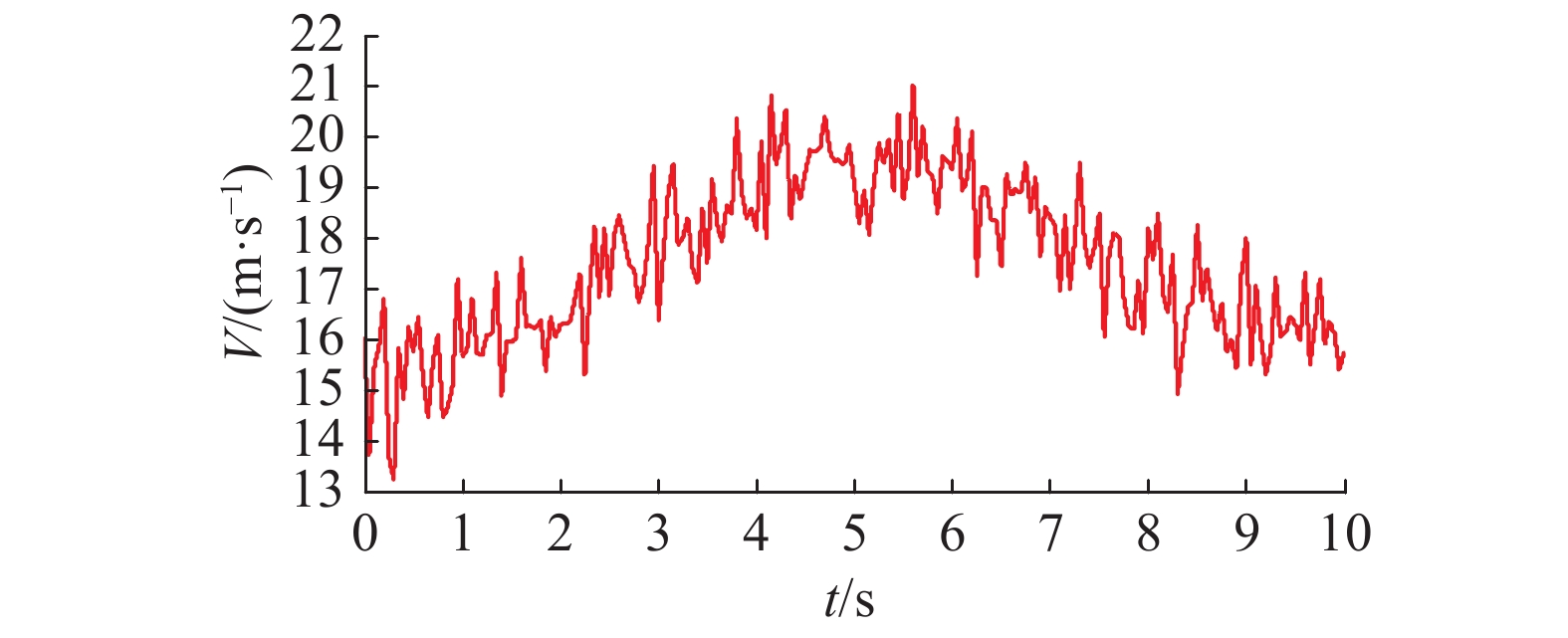Download: 图 12 风速 Fig. 12 The curve of wind speed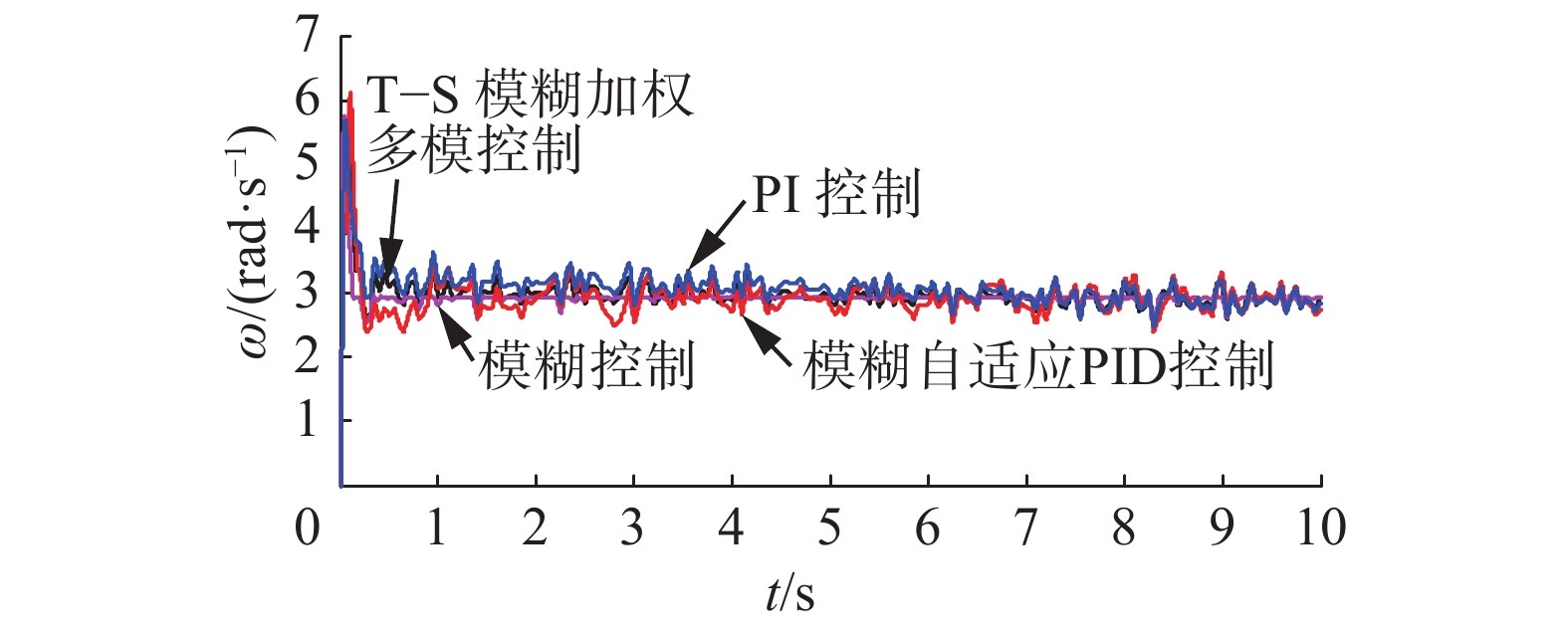Download: 图 13 转速对比 Fig. 13 The comparison of rotor speed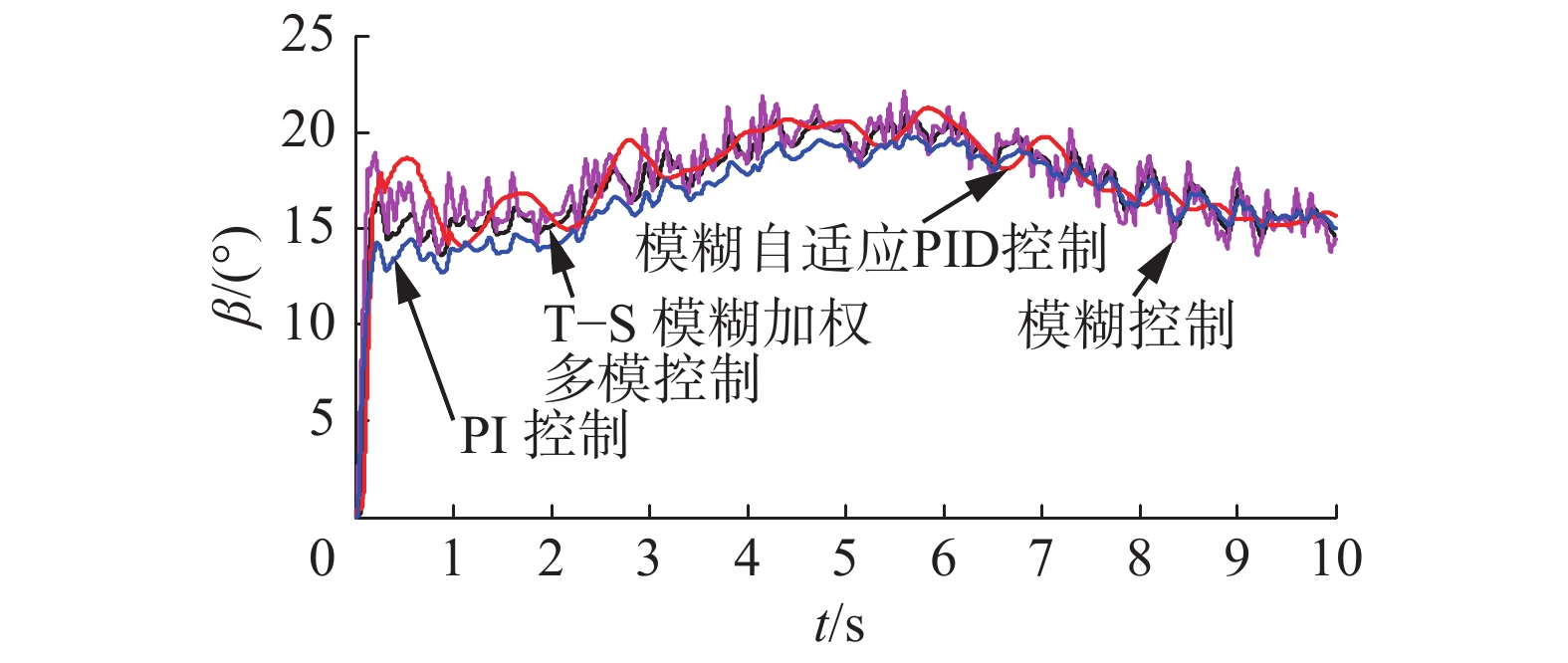Download: 图 14 桨距角对比 Fig. 14 The comparison of pitch angle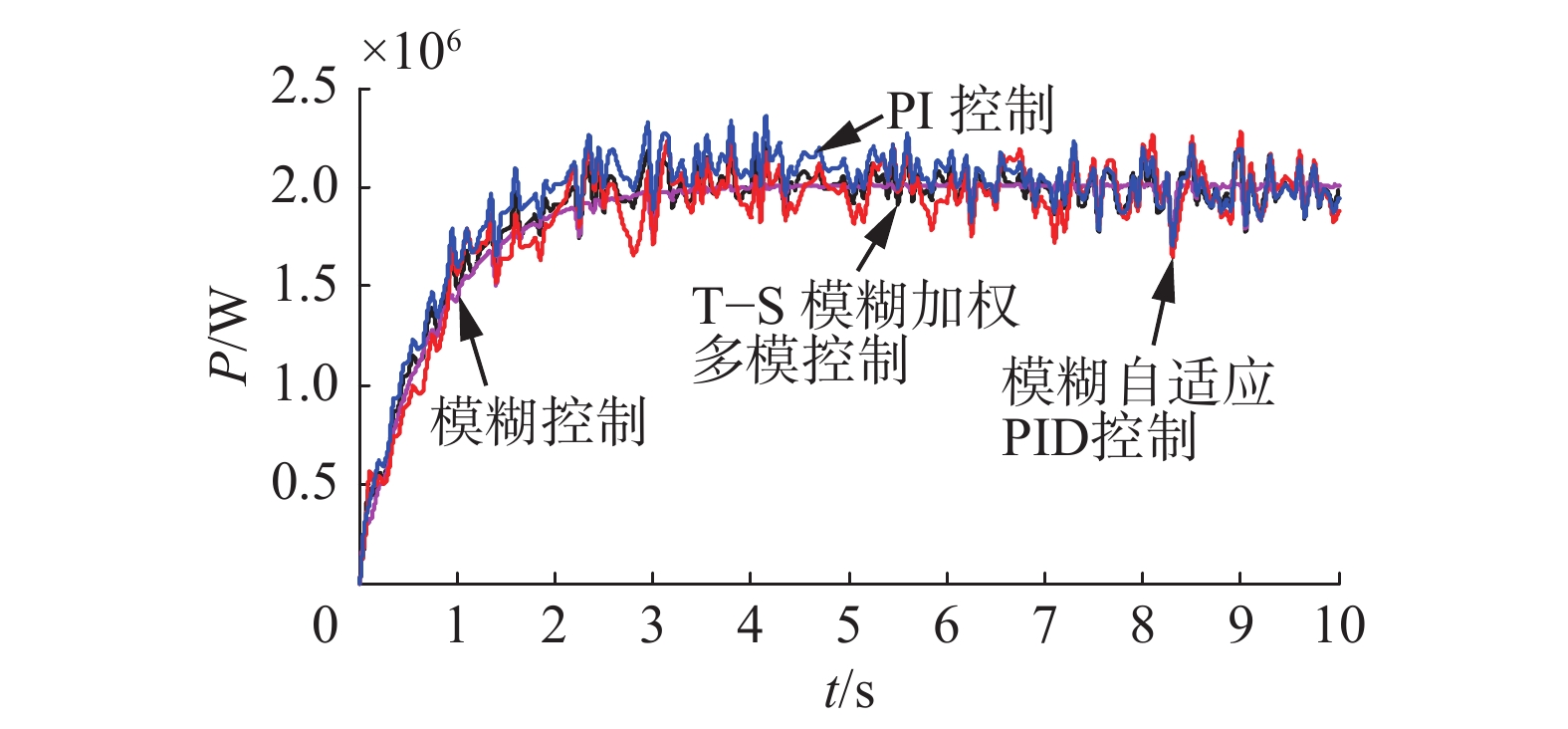Download: 图 15 输出功率对比 Fig. 15 The comparison of generator output power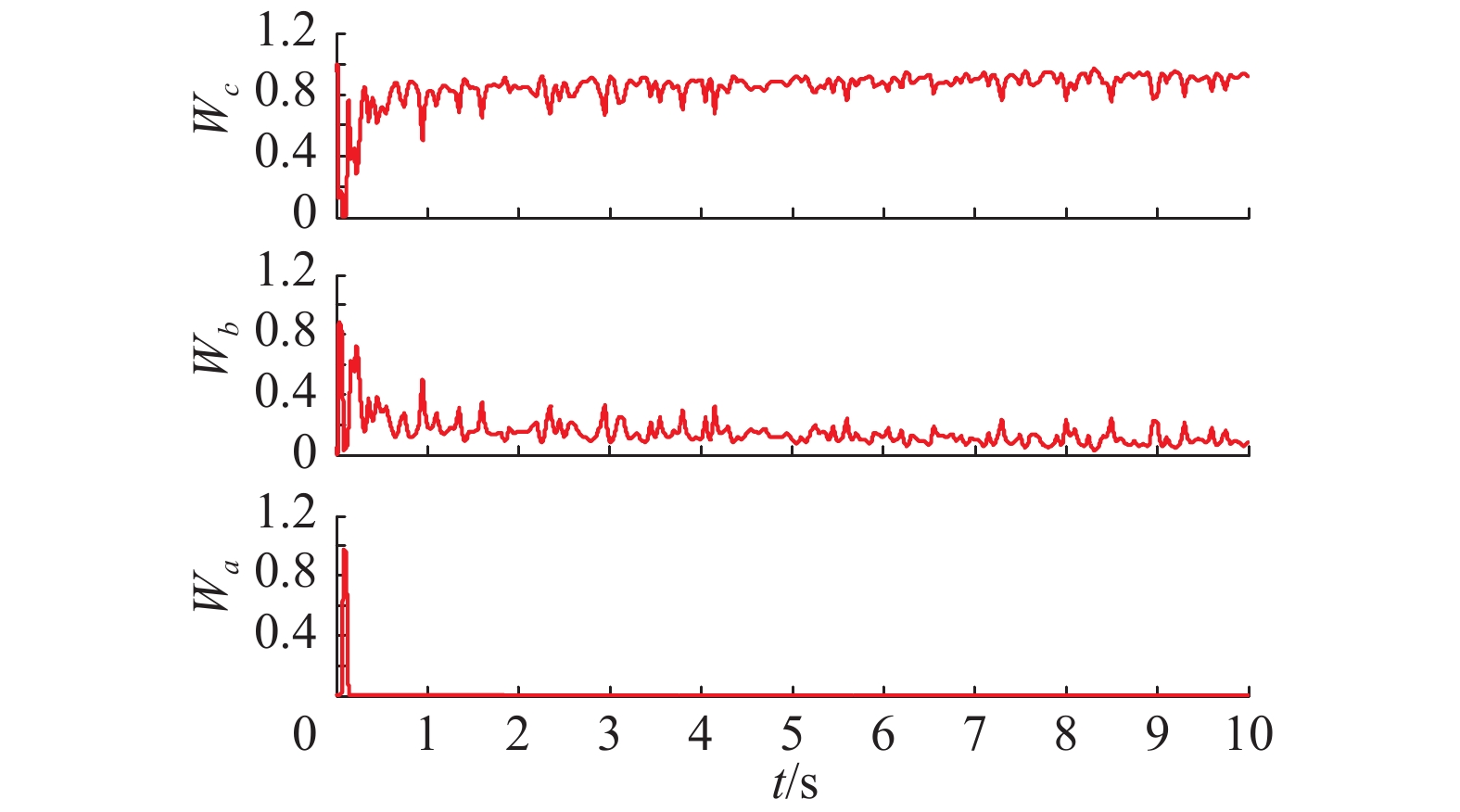Download: 图 16 T-S模糊推理的权值输出 Fig. 16 The weight output of T-S fuzzy inference

5 结束语

  YIN Xiuxing, LIN Yonggang, LI Wei, et al. Adaptive sliding mode back-stepping pitch angle control of a variable-displacement pump controlled pitch system for wind turbines[J]. ISA transactions, 2015, 58: 629-634. DOI:10.1016/j.isatra.2015.07.006 (0)  YUAN Yuan, TANG J. Adaptive pitch control of wind turbine for load mitigation under structural uncertainties[J]. Renewable energy, 2017, 105: 483-494. DOI:10.1016/j.renene.2016.12.068 (0)  SONG Dongran, YANG Jian, DONG Mi, et al. Model predictive control with finite control set for variable-speed wind turbines[J]. Energy, 2017, 126: 564-572. DOI:10.1016/j.energy.2017.02.149 (0)  吴洁, 孟克其劳, 贾大江, 等. 1.5MW永磁直驱风力发电机组变桨控制系统的改进[J]. 太阳能学报, 2016, 37(8): 2074-2079. WU Jie, MENG Keqilao, JIA Dajiang, et al. Improved pitch control system of 1.5 MW permanent magnet direct drive wind turbine[J]. Atct energiae solaris sinica, 2016, 37(8): 2074-2079. (0)  MORADI H, VOSSOUGHI G. Robust control of the variable speed wind turbines in the presence of uncertainties: a comparison between H∞, and PID controllers [J]. Energy, 2015, 90: 1508-1521. DOI:10.1016/j.energy.2015.06.100 (0)  任丽娜, 吕明月, 王振臣. 改进蜂群算法在风机变桨距控制中的应用[J]. 机械设计与制造, 2015, 36(3): 43-46. REN Lina, LV Mingyue, WANG Zhenchen. Improved bee colony algorithm on the wind turbine pitch control[J]. Machinery design and manufacture, 2015, 36(3): 43-46. (0)  Yin Xiuxing, Lin Yonggang, Li Wei, et al. A novel fuzzy integral sliding mode current control strategy for maximizing wind power extraction and eliminating voltage harmonics[J]. Energy, 2015, 85: 677-686. DOI:10.1016/j.energy.2015.04.005 (0)  WANG Baohua, Qin Shengsheng. Backstepping sliding mode control of variable pitch wind power system[C]//2010 Asia-Pacific Power and Energy Engineering Conference (APPEEC). Chengdu, China, 2010: 1–3. (0)  MEDJBER A, GUESSOUM A, BELMILI H, et al. New neural network and fuzzy logic controllers to monitor maximum power for wind energy conversion system[J]. Energy, 2016, 106: 137-146. DOI:10.1016/j.energy.2016.03.026 (0)  QIN Bin, ZHOU Hao, DU Kang, et al. Sliding mode control of pitch angle based on RBF neural-network[J]. Transactions of China electrotechnical society, 2013, 28(5): 37-41. (0)  周飞航, 刘军. 基于状态反馈的直驱风电系统模糊控制策略[J]. 电网技术, 2016, 40(9): 2758-2763. ZHOU Feihang, LIU Jun. Fuzzy control strategy of direct-drive wind power system based on state feedback[J]. Power system technology, 2016, 40(9): 2758-2763. (0)  ANDERSON P M, BOSE A. Stability simulation of wind turbine systems[J]. IEEE transactions on power apparatus and systems, 1983, PAS-102(12): 3791-3795. DOI:10.1109/TPAS.1983.317873 (0)  BOUKHEZZAR B, LUPU L, SIGUERDIDJANE H, et al. Multivariable control strategy for variable speed, variable pitch wind turbines[J]. Renewable energy, 2007, 32(8): 1273-1287. DOI:10.1016/j.renene.2006.06.010 (0)  HUI J, BAKHSHAI A. A new adaptive control algorithm for maximum power point tracking for wind energy conversion systems[C]//2008 PESC Proceedings of the Power Electronics Specialist Conference. Rhodes, Greece, 2008: 4003–4007. (0)  DAHBI A, NAIT-SAID N, NAIT-SAID M-S. A novel combined MPPT-pitch angle control for wide range variable speed wind turbine based on neural network[J]. International journal of hydrogen energy, 2016, 41(22): 9427-9442. DOI:10.1016/j.ijhydene.2016.03.105 (0)  ZHANG Xinfang, XU Daping. Adaptive fuzzy control for variable speed variable pitch wind turbines[J]. IFAC power plants and power systems control, 2003, 36(20): 1031-1036. (0)  BAGHERI P, Sun Qiao. Adaptive robust control of a class of non-affine variable-speed variable-pitch wind turbines with unmodeled dynamics[J]. ISA transactions, 2016, 63: 233-241. DOI:10.1016/j.isatra.2016.04.008 (0)  唐酿, 肖湘宁, 陈众. 基于Sugeno模糊推理的静止无功补偿器多模态切换方法[J]. 电网技术, 2011, 35(8): 140-143. TANG Niang, XIAO Xiangning, CHEN Zhong. A method of multi-mode switching for SVC based on sugeno fuzzy inference[J]. Power system technology, 2011, 35(8): 140-143. (0)